# Zoom levels and tile grid

Azure Maps use the Spherical Mercator projection coordinate system (EPSG: 3857). A projection is the mathematical model used to transform the spherical globe into a flat map. The Spherical Mercator projection stretches the map at the poles to create a square map. This projection significantly distorts the scale and area of the map but has two important properties that outweigh this distortion:

• It's a conformal projection, which means that it preserves the shape of relatively small objects. Preserving the shape of small objects is especially important when showing aerial imagery. For example, we want to avoid distorting the shape of buildings. Square buildings should appear square, not rectangular.
• It's a cylindrical projection. North and south are always up and down, and west and east are always left and right.

To optimize the performance of map retrieval and display, the map is divided into square tiles. The Azure Maps SDK's use tiles that have a size of 512 x 512 pixels for road maps, and smaller 256 x 256 pixels for satellite imagery. Azure Maps provides raster and vector tiles for 23 zoom levels, numbered 0 through 22. At zoom level 0, the entire world fits on a single tile: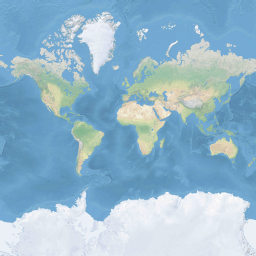Zoom level 1 uses four tiles to render the world: a 2 x 2 square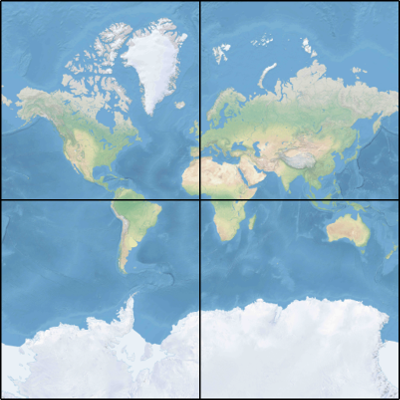Each additional zoom level quad-divides the tiles of the previous one, creating a grid of 2zoom x 2zoom. Zoom level 22 is a grid 222 x 222, or 4,194,304 x 4,194,304 tiles (17,592,186,044,416 tiles in total).

The Azure Maps interactive map controls for web and Android support 25 zoom levels, numbered 0 through 24. Although road data will only be available at the zoom levels in when the tiles are available.

The following table provides the full list of values for zoom levels where the tile size is 512 pixels square at latitude 0:

Zoom level Meters/pixel Meters/tile side
0 156543 40075017
1 78271.5 20037508
2 39135.8 10018754
3 19567.88 5009377.1
4 9783.94 2504688.5
5 4891.97 1252344.3
6 2445.98 626172.1
7 1222.99 313086.1
8 611.5 156543
9 305.75 78271.5
10 152.87 39135.8
11 76.44 19567.9
12 38.219 9783.94
13 19.109 4891.97
14 9.555 2445.98
15 4.777 1222.99
16 2.3887 611.496
17 1.1943 305.748
18 0.5972 152.874
19 0.2986 76.437
20 0.14929 38.2185
21 0.074646 19.10926
22 0.037323 9.55463
23 0.0186615 4.777315
24 0.00933075 2.3886575

## Pixel coordinates

Having chosen the projection and scale to use at each zoom level, we can convert geographic coordinates into pixel coordinates. The full pixel width and height of a map image of the world for a particular zoom level is calculated as:

``````var mapWidth = tileSize * Math.pow(2, zoom);

var mapHeight = mapWidth;
``````

Since the map width and height is different at each zoom level, so are the pixel coordinates. The pixel at the upper-left corner of the map always has pixel coordinates (0, 0). The pixel at the lower-right corner of the map has pixel coordinates (width-1, height-1), or referring to the equations in the previous section, (tileSize * 2zoom–1, tileSize * 2zoom–1). For example, when using 512 square tiles at level 2, the pixel coordinates range from (0, 0) to (2047, 2047), like this: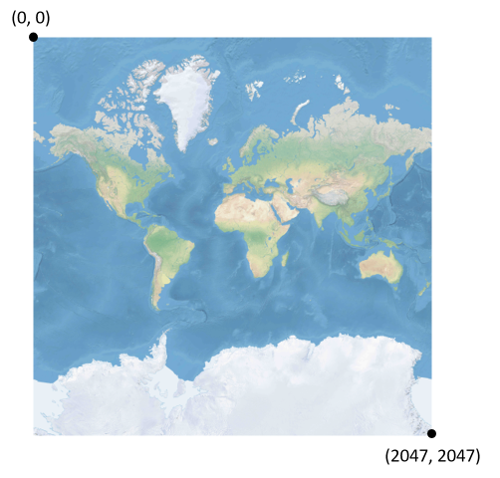Given latitude and longitude in degrees, and the level of detail, the pixel XY coordinates is calculated as follows:

``````var sinLatitude = Math.sin(latitude * Math.PI/180);

var pixelX = ((longitude + 180) / 360) * tileSize * Math.pow(2, zoom);

var pixelY = (0.5 – Math.log((1 + sinLatitude) / (1 – sinLatitude)) / (4 * Math.PI)) * tileSize * Math.pow(2, zoom);
``````

The latitude and longitude values are assumed to be on the WGS 84 datum. Even though Azure Maps uses a spherical projection, it's important to convert all geographic coordinates into a common datum. WGS 84 is the selected datum. The longitude value is assumed to range from -180 degrees to +180 degrees, and the latitude value must be clipped to range from -85.05112878 to 85.05112878. Adhering to these values avoids a singularity at the poles, and it ensures that the projected map is a squared shape.

## Tile coordinates

To optimize the performance of map retrieval and display, the rendered map is cut into tiles. The number of pixels and the number of tiles differ at each zoom level:

``````var numberOfTilesWide = Math.pow(2, zoom);

var numberOfTilesHigh = numberOfTilesWide;
``````

Each tile is given XY coordinates ranging from (0, 0) in the upper left to (2zoom–1, 2zoom–1) in the lower right. For example, at zoom level 3, the tile coordinates range from (0, 0) to (7, 7) as follows: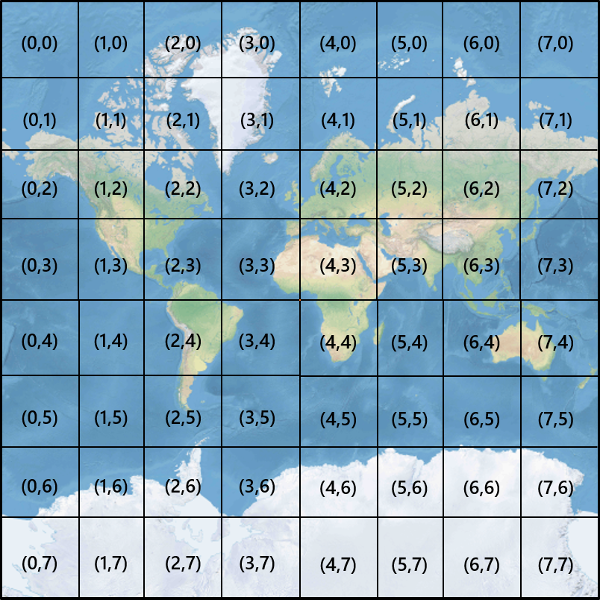Given a pair of pixel XY coordinates, you can easily determine the tile XY coordinates of the tile containing that pixel:

``````var tileX = Math.floor(pixelX / tileSize);

var tileY = Math.floor(pixelY / tileSize);
``````

Tiles are called by zoom level. The x and y coordinates correspond to the tile's position on the grid for that zoom level.

When determining which zoom level to use, remember each location is in a fixed position on its tile. As a result, the number of tiles needed to display a given expanse of territory is dependent on the specific placement of zoom grid on the world map. For instance, if there are two points 900 meters apart, it may only take three tiles to display a route between them at zoom level 17. However, if the western point is on the right of its tile, and the eastern point on the left of its tile, it may take four tiles: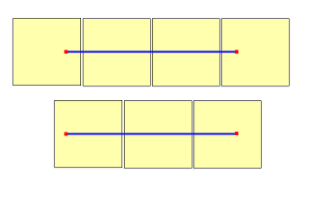Once the zoom level is determined, the x and y values can be calculated. The top-left tile in each zoom grid is x=0, y=0; the bottom-right tile is at x=2zoom-1, y=2zoom-1.

Here is the zoom grid for zoom level 1:Some mapping platforms use a `quadkey` indexing naming convention that combines the tile ZY coordinates into a one-dimension string called `quadtree` keys or `quadkeys` for short. Each `quadkey` uniquely identifies a single tile at a particular level of detail, and it can be used as a key in common database B-tree indexes. The Azure Maps SDKs support the overlaying of tile layers that use `quadkey` naming convention in addition to other naming conventions as documented in the Add a tile layer document.

Note

The `quadkeys` naming convention only works for zoom levels of one or greater. The Azure Maps SDK's support zoom level 0 which is a single map tile for the whole world.

To convert tile coordinates into a `quadkey`, the bits of the Y and X coordinates are interleaved, and the result is interpreted as a base-4 number (with leading zeros maintained) and converted into a string. For instance, given tile XY coordinates of (3, 5) at level 3, the `quadkey` is determined as follows:

``````tileX = 3 = 011 (base 2)

tileY = 5 = 101 (base 2)

quadkey = 100111 (base 2) = 213 (base 4) = "213"
``````

`Qquadkeys` have several interesting properties. First, the length of a `quadkey` (the number of digits) equals the zoom level of the corresponding tile. Second, the `quadkey` of any tile starts with the `quadkey` of its parent tile (the containing tile at the previous level). As shown in the example below, tile 2 is the parent of tiles 20 through 23:Finally, `quadkeys` provide a one-dimensional index key that usually preserves the proximity of tiles in XY space. In other words, two tiles that have nearby XY coordinates usually have `quadkeys` that are relatively close together. This is important for optimizing database performance, because neighboring tiles are often requested in groups, and it's desirable to keep those tiles on the same disk blocks, in order to minimize the number of disk reads.

## Tile math source code

The following sample code illustrates how to implement the functions described in this document. These functions can be easily translated into other programming languages as needed.

``````using System;
using System.Text;

namespace AzureMaps
{
/// <summary>
/// Tile System math for the Spherical Mercator projection coordinate system (EPSG:3857)
/// </summary>
public static class TileMath
{
private const double EarthRadius = 6378137;

private const double MinLatitude = -85.05112878;
private const double MaxLatitude = 85.05112878;
private const double MinLongitude = -180;
private const double MaxLongitude = 180;

/// <summary>
/// Clips a number to the specified minimum and maximum values.
/// </summary>
/// <param name="n">The number to clip.</param>
/// <param name="minValue">Minimum allowable value.</param>
/// <param name="maxValue">Maximum allowable value.</param>
/// <returns>The clipped value.</returns>
private static double Clip(double n, double minValue, double maxValue)
{
return Math.Min(Math.Max(n, minValue), maxValue);
}

/// <summary>
/// Calculates width and height of the map in pixels at a specific zoom level from -180 degrees to 180 degrees.
/// </summary>
/// <param name="zoom">Zoom Level to calculate width at</param>
/// <param name="tileSize">The size of the tiles in the tile pyramid.</param>
/// <returns>Width and height of the map in pixels</returns>
public static double MapSize(double zoom, int tileSize)
{
return Math.Ceiling(tileSize * Math.Pow(2, zoom));
}

/// <summary>
/// Calculates the Ground resolution at a specific degree of latitude in meters per pixel.
/// </summary>
/// <param name="latitude">Degree of latitude to calculate resolution at</param>
/// <param name="zoom">Zoom level to calculate resolution at</param>
/// <param name="tileSize">The size of the tiles in the tile pyramid.</param>
/// <returns>Ground resolution in meters per pixels</returns>
public static double GroundResolution(double latitude, double zoom, int tileSize)
{
latitude = Clip(latitude, MinLatitude, MaxLatitude);
return Math.Cos(latitude * Math.PI / 180) * 2 * Math.PI * EarthRadius / MapSize(zoom, tileSize);
}

/// <summary>
/// Determines the map scale at a specified latitude, level of detail, and screen resolution.
/// </summary>
/// <param name="latitude">Latitude (in degrees) at which to measure the map scale.</param>
/// <param name="zoom">Level of detail, from 1 (lowest detail) to 23 (highest detail).</param>
/// <param name="screenDpi">Resolution of the screen, in dots per inch.</param>
/// <param name="tileSize">The size of the tiles in the tile pyramid.</param>
/// <returns>The map scale, expressed as the denominator N of the ratio 1 : N.</returns>
public static double MapScale(double latitude, double zoom, int screenDpi, int tileSize)
{
return GroundResolution(latitude, zoom, tileSize) * screenDpi / 0.0254;
}

/// <summary>
/// Global Converts a Pixel coordinate into a geospatial coordinate at a specified zoom level.
/// Global Pixel coordinates are relative to the top left corner of the map (90, -180)
/// </summary>
/// <param name="pixel">Pixel coordinates in the format of [x, y].</param>
/// <param name="zoom">Zoom level</param>
/// <param name="tileSize">The size of the tiles in the tile pyramid.</param>
/// <returns>A position value in the format [longitude, latitude].</returns>
public static double[] GlobalPixelToPosition(double[] pixel, double zoom, int tileSize)
{
var mapSize = MapSize(zoom, tileSize);

var x = (Clip(pixel, 0, mapSize - 1) / mapSize) - 0.5;
var y = 0.5 - (Clip(pixel, 0, mapSize - 1) / mapSize);

return new double[] {
360 * x,    //Longitude
90 - 360 * Math.Atan(Math.Exp(-y * 2 * Math.PI)) / Math.PI  //Latitude
};
}

/// <summary>
/// Converts a point from latitude/longitude WGS-84 coordinates (in degrees) into pixel XY coordinates at a specified level of detail.
/// </summary>
/// <param name="position">Position coordinate in the format [longitude, latitude]</param>
/// <param name="zoom">Zoom level.</param>
/// <param name="tileSize">The size of the tiles in the tile pyramid.</param>
/// <returns>A global pixel coordinate.</returns>
public static double[] PositionToGlobalPixel(double[] position, int zoom, int tileSize)
{
var latitude = Clip(position, MinLatitude, MaxLatitude);
var longitude = Clip(position, MinLongitude, MaxLongitude);

var x = (longitude + 180) / 360;
var sinLatitude = Math.Sin(latitude * Math.PI / 180);
var y = 0.5 - Math.Log((1 + sinLatitude) / (1 - sinLatitude)) / (4 * Math.PI);

var mapSize = MapSize(zoom, tileSize);

return new double[] {
Clip(x * mapSize + 0.5, 0, mapSize - 1),
Clip(y * mapSize + 0.5, 0, mapSize - 1)
};
}

/// <summary>
/// Converts pixel XY coordinates into tile XY coordinates of the tile containing the specified pixel.
/// </summary>
/// <param name="pixel">Pixel coordinates in the format of [x, y].</param>
/// <param name="tileSize">The size of the tiles in the tile pyramid.</param>
/// <param name="tileX">Output parameter receiving the tile X coordinate.</param>
/// <param name="tileY">Output parameter receiving the tile Y coordinate.</param>
public static void GlobalPixelToTileXY(double[] pixel, int tileSize, out int tileX, out int tileY)
{
tileX = (int)(pixel / tileSize);
tileY = (int)(pixel / tileSize);
}

/// <summary>
/// Performs a scale transform on a global pixel value from one zoom level to another.
/// </summary>
/// <param name="pixel">Pixel coordinates in the format of [x, y].</param>
/// <param name="oldZoom">The zoom level in which the input global pixel value is from.</param>
/// <returns>A scale pixel coordinate.</returns>
public static double[] ScaleGlobalPixel(double[] pixel, double oldZoom, double newZoom)
{
var scale = Math.Pow(2, oldZoom - newZoom);

return new double[] { pixel * scale, pixel * scale };
}

/// <summary>
/// Performs a scale transform on a set of global pixel values from one zoom level to another.
/// </summary>
/// <param name="pixels">A set of global pixel value from the old zoom level. Points are in the format [x,y].</param>
/// <param name="oldZoom">The zoom level in which the input global pixel values is from.</param>
/// <param name="newZoom">The new zoom level in which the output global pixel values should be aligned with.</param>
/// <returns>A set of global pixel values that has been scaled for the new zoom level.</returns>
public static double[][] ScaleGlobalPixels(double[][] pixels, double oldZoom, double newZoom)
{
var scale = Math.Pow(2, oldZoom - newZoom);

var output = new System.Collections.Generic.List<double[]>();
foreach (var p in pixels)
{
output.Add(new double[] { p * scale, p * scale });
}

return output.ToArray();
}

/// <summary>
/// Converts tile XY coordinates into a global pixel XY coordinates of the upper-left pixel of the specified tile.
/// </summary>
/// <param name="tileX">Tile X coordinate.</param>
/// <param name="tileY">Tile Y coordinate.</param>
/// <param name="tileSize">The size of the tiles in the tile pyramid.</param>
/// <param name="pixelX">Output parameter receiving the X coordinate of the point, in pixels.</param>
/// <param name="pixelY">Output parameter receiving the Y coordinate of the point, in pixels.</param>
public static double[] TileXYToGlobalPixel(int tileX, int tileY, int tileSize)
{
return new double[] { tileX * tileSize, tileY * tileSize };
}

/// <summary>
/// Converts tile XY coordinates into a quadkey at a specified level of detail.
/// </summary>
/// <param name="tileX">Tile X coordinate.</param>
/// <param name="tileY">Tile Y coordinate.</param>
/// <param name="zoom">Zoom level</param>
/// <returns>A string containing the quadkey.</returns>
public static string TileXYToQuadKey(int tileX, int tileY, int zoom)
{
for (int i = zoom; i > 0; i--)
{
char digit = '0';
int mask = 1 << (i - 1);
if ((tileX & mask) != 0)
{
digit++;
}
if ((tileY & mask) != 0)
{
digit++;
digit++;
}
}
}

/// <summary>
/// Converts a quadkey into tile XY coordinates.
/// </summary>
/// <param name="tileX">Output parameter receiving the tile X coordinate.</param>
/// <param name="tileY">Output parameter receiving the tile Y coordinate.</param>
/// <param name="zoom">Output parameter receiving the zoom level.</param>
public static void QuadKeyToTileXY(string quadKey, out int tileX, out int tileY, out int zoom)
{
tileX = tileY = 0;
for (int i = zoom; i > 0; i--)
{
int mask = 1 << (i - 1);
{
case '0':
break;

case '1':
break;

case '2':
break;

case '3':
break;

default:
throw new ArgumentException("Invalid QuadKey digit sequence.");
}
}
}

/// <summary>
/// Calculates the XY tile coordinates that a coordinate falls into for a specific zoom level.
/// </summary>
/// <param name="position">Position coordinate in the format [longitude, latitude]</param>
/// <param name="zoom">Zoom level</param>
/// <param name="tileSize">The size of the tiles in the tile pyramid.</param>
/// <param name="tileX">Output parameter receiving the tile X position.</param>
/// <param name="tileY">Output parameter receiving the tile Y position.</param>
public static void PositionToTileXY(double[] position, int zoom, int tileSize, out int tileX, out int tileY)
{
var latitude = Clip(position, MinLatitude, MaxLatitude);
var longitude = Clip(position, MinLongitude, MaxLongitude);

var x = (longitude + 180) / 360;
var sinLatitude = Math.Sin(latitude * Math.PI / 180);
var y = 0.5 - Math.Log((1 + sinLatitude) / (1 - sinLatitude)) / (4 * Math.PI);

//tileSize needed in calculations as in rare cases the multiplying/rounding/dividing can make the difference of a pixel which can result in a completely different tile.
var mapSize = MapSize(zoom, tileSize);
tileX = (int)Math.Floor(Clip(x * mapSize + 0.5, 0, mapSize - 1) / tileSize);
tileY = (int)Math.Floor(Clip(y * mapSize + 0.5, 0, mapSize - 1) / tileSize);
}

/// <summary>
/// Calculates the tile quadkey strings that are within a specified viewport.
/// </summary>
/// <param name="position">Position coordinate in the format [longitude, latitude]</param>
/// <param name="zoom">Zoom level</param>
/// <param name="width">The width of the map viewport in pixels.</param>
/// <param name="height">The height of the map viewport in pixels.</param>
/// <param name="tileSize">The size of the tiles in the tile pyramid.</param>
/// <returns>A list of quadkey strings that are within the specified viewport.</returns>
public static string[] GetQuadkeysInView(double[] position, int zoom, int width, int height, int tileSize)
{
var p = PositionToGlobalPixel(position, zoom, tileSize);

var top = p - height * 0.5;
var left = p - width * 0.5;

var bottom = p + height * 0.5;
var right = p + width * 0.5;

var tl = GlobalPixelToPosition(new double[] { left, top }, zoom, tileSize);
var br = GlobalPixelToPosition(new double[] { right, bottom }, zoom, tileSize);

//Boudning box in the format: [west, south, east, north];
var bounds = new double[] { tl, br, br, tl };

}

/// <summary>
/// Calculates the tile quadkey strings that are within a bounding box at a specific zoom level.
/// </summary>
/// <param name="bounds">A bounding box defined as an array of numbers in the format of [west, south, east, north].</param>
/// <param name="zoom">Zoom level to calculate tiles for.</param>
/// <param name="tileSize">The size of the tiles in the tile pyramid.</param>
/// <returns>A list of quadkey strings.</returns>
public static string[] GetQuadkeysInBoundingBox(double[] bounds, int zoom, int tileSize)
{
var keys = new System.Collections.Generic.List<string>();

if (bounds != null && bounds.Length >= 4)
{
PositionToTileXY(new double[] { bounds, bounds }, zoom, tileSize, out int tlX, out int tlY);
PositionToTileXY(new double[] { bounds, bounds }, zoom, tileSize, out int brX, out int brY);

for (int x = tlX; x <= brX; x++)
{
for (int y = tlY; y <= brY; y++)
{
}
}
}

return keys.ToArray();
}

/// <summary>
/// Calculates the bounding box of a tile.
/// </summary>
/// <param name="tileX">Tile X coordinate</param>
/// <param name="tileY">Tile Y coordinate</param>
/// <param name="zoom">Zoom level</param>
/// <param name="tileSize">The size of the tiles in the tile pyramid.</param>
/// <returns>A bounding box of the tile defined as an array of numbers in the format of [west, south, east, north].</returns>
public static double[] TileXYToBoundingBox(int tileX, int tileY, double zoom, int tileSize)
{
//Top left corner pixel coordinates
var x1 = (double)(tileX * tileSize);
var y1 = (double)(tileY * tileSize);

//Bottom right corner pixel coordinates
var x2 = (double)(x1 + tileSize);
var y2 = (double)(y1 + tileSize);

var nw = GlobalPixelToPosition(new double[] { x1, y1 }, zoom, tileSize);
var se = GlobalPixelToPosition(new double[] { x2, y2 }, zoom, tileSize);

return new double[] { nw, se, se, nw };
}

/// <summary>
/// Calculates the best map view (center, zoom) for a bounding box on a map.
/// </summary>
/// <param name="bounds">A bounding box defined as an array of numbers in the format of [west, south, east, north].</param>
/// <param name="mapWidth">Map width in pixels.</param>
/// <param name="mapHeight">Map height in pixels.</param>
/// <param name="padding">Width in pixels to use to create a buffer around the map. This is to keep markers from being cut off on the edge</param>
/// <param name="tileSize">The size of the tiles in the tile pyramid.</param>
/// <param name="latitude">Output parameter receiving the center latitude coordinate.</param>
/// <param name="longitude">Output parameter receiving the center longitude coordinate.</param>
/// <param name="zoom">Output parameter receiving the zoom level</param>
public static void BestMapView(double[] bounds, double mapWidth, double mapHeight, int padding, int tileSize, out double centerLat, out double centerLon, out double zoom)
{
if (bounds == null || bounds.Length < 4)
{
centerLat = 0;
centerLon = 0;
zoom = 1;
return;
}

double boundsDeltaX;

//Check if east value is greater than west value which would indicate that bounding box crosses the antimeridian.
if (bounds > bounds)
{
boundsDeltaX = bounds - bounds;
centerLon = (bounds + bounds) / 2;
}
else
{
boundsDeltaX = 360 - (bounds - bounds);
centerLon = ((bounds + bounds) / 2 + 360) % 360 - 180;
}

var ry1 = Math.Log((Math.Sin(bounds * Math.PI / 180) + 1) / Math.Cos(bounds * Math.PI / 180));
var ry2 = Math.Log((Math.Sin(bounds * Math.PI / 180) + 1) / Math.Cos(bounds * Math.PI / 180));
var ryc = (ry1 + ry2) / 2;

centerLat = Math.Atan(Math.Sinh(ryc)) * 180 / Math.PI;

var resolutionHorizontal = boundsDeltaX / (mapWidth - padding * 2);

var vy0 = Math.Log(Math.Tan(Math.PI * (0.25 + centerLat / 360)));
var vy1 = Math.Log(Math.Tan(Math.PI * (0.25 + bounds / 360)));
var zoomFactorPowered = (mapHeight * 0.5 - padding) / (40.7436654315252 * (vy1 - vy0));
var resolutionVertical = 360.0 / (zoomFactorPowered * tileSize);

var resolution = Math.Max(resolutionHorizontal, resolutionVertical);

zoom = Math.Log(360 / (resolution * tileSize), 2);
}
}
}
``````

Note

The interactive map controls in the Azure Maps SDK's have helper functions for converting between geospatial positions and viewport pixels.

## Next steps

Directly access map tiles from the Azure Maps REST services: